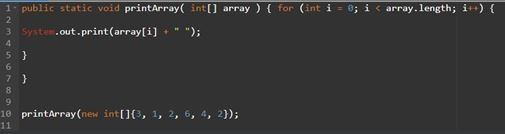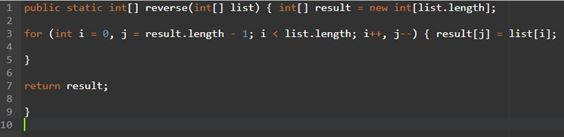Our Special Offer - Get 3 Courses at 24,999/- Only. Read more
Hire Talent (HR):+91-9707 240 250

# General# Java Array

## Java Array

An array is collection of objects and all the objects have the same name. Each object is called an element. The elements are numbered as 0, 1, 2,…, n-1. These numbers are called as indices or subscripts. These numbers are used to locate the positions of elements within the array.

The method of numbering the ith element with index i-1 is called as zero-based indexing. That is, the element is always same as the number of “steps” from the initial element a to that element. For example, the element a is 3 steps from the element a.

Array can Strore data in index based and it can store only homogeneous data. Once we create an array we cannot chage the array size (increase or decrease) at run time. we can retrieve or store the data based on index and we can store huge data with the same name.Readability is easy.

Example:

```Int[] array=new int;

Int[] array={10,20,30,40,40};```

In the above mentioned ways we can create arrays.

array – it will return 10.because 0 th index 10 value is there.

### Types of arrays

There are three types of arrays.

• One-dimensional array
• Two-dimensional array
• Multi-dimensional array

### One-dimensional array

It is an array in which each element is accessed by using only one subscript. The only one subscript represents the position of the element in the array.

#### Declaration of one-dimensional array

Syntax:

`datatype    array-name[size];`

Example :

` int     marks;`

#### Initialization of one-dimensional arrays

We can give values to each array element when the array is first defined.

Example :

`int a = {9, -5, 6, 2, 8};`

In the above example, value 9 is stored in a, value -5 is stored in a, value 6 is store in a, value 2 is stored in a and value 8 is store in a.

#### Memory representation of one-dimensional arrays:

The elements of one-dimensional arrays are stored in contiguous memory locations.

Example:

`Consider the declaration,   char a;`

The element a is allocated at a particular memory location, the element a is allocated at next memory location and so forth. Since the array is of the type char, each element requires 1-byte.

### Multi-dimensional array

A multidimensional array is an array of n-dimensions. In other words, an array of arrays is called a multidimensional array. A one-dimensional array of one-dimensional arrays is called a two-dimensional array; a one-dimensional array to two-dimensional arrays is called a three-dimensional array and so on.

#### Three steps to create an array:

• Declare a variable to hold the array.
• Create a new array object and assign it to the array variable.
• Store things in that array.

Example:

```String[] names;
names = new String;
names  = “n1”;
names = ‘n2’;

. . .

```

Java does not support multidimensional arrays. However, we can declare and create an array of arrays and access them as you would C-style multidimensional arrays.

### Creating Arrays

For create an array we can use the new operator with the below syntax:

`Array RefVar = new dataType[arraySize];`

#### Passing Array to Methods

Just as we can pass original type values to methods, We can also pass arrays to methods.#### Returning an Array from Methods

This method shown below returns an array that is the reversal of another array:### The Array Class

The java.util.Arrays class contains different static strategies for arranging and seeking arrays, looking at arrays, and filling array components. These strategies are over-burden for every crude sort.

#### public static int binary Search(Object[] an, Object key)

Ventures the predefined array of Object ( Byte, Int , twofold, and so on.) for the predetermined esteem utilizing the double pursuit calculation. The array must be arranged preceding making this call. This profits file of the pursuit key, on the off chance that it is contained in the rundown; generally, (‐(insertion point + 1).

#### public static Boolean equals(long[] a, long[] a2)

Returns genuine if the two indicated arrays of yearns are equivalent to each other. Two arrays are viewed as equivalent if both arrays contain a similar number of components, and every single comparing pair of components in the two arrays are equivalent. This profits genuine if the two arrays are equivalent. Same technique could be utilized by all other crude information types (Byte, short, Int, and so on.)

#### public static void fill(int[] an, int val)

Doles out the predetermined int incentive to every component of the predefined array of ints. Same strategy could be utilized by all other crude information types (Byte, short, Int and so forth.)

#### public static void sort(Object[] a)

Sorts the predetermined array of items into rising request, as indicated by the common requesting of its components. Same strategy could be utilized by all other crude information types ( Byte, short, Int, and so on.)